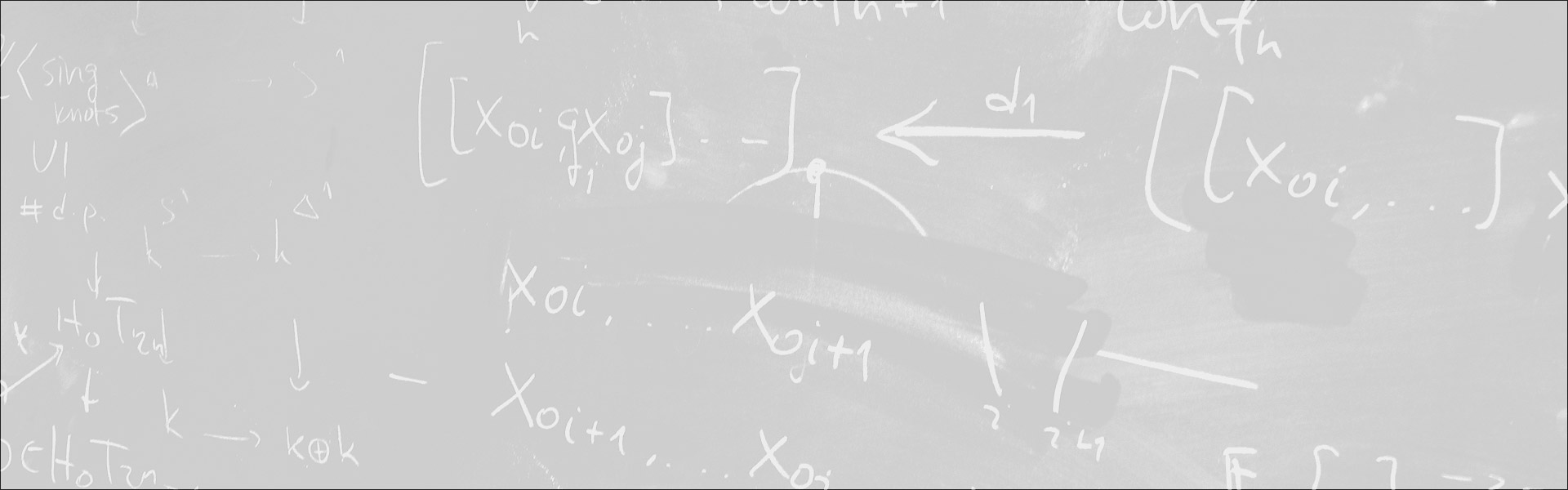Designed and built with care, filled with creative elements

Top# Linearization procedures in the semi-minimal analysis of algebraic differential equations

/  Évènements• Cet évènement est passé

## Linearization procedures in the semi-minimal analysis of algebraic differential equations

It is well-known that certain algebraic differential equations restrain in an essential way the algebraic relations that their solutions share. For example, the solutions of the first equation of Painlevé y » = 6y^2 + t are “new” transcendental functions of order two which whenever distinct are algebraically independent (together with their derivatives).
I will first describe an account of such phenomena using the language of geometric stability theory in a differentially closed field. I will then explain how linearization procedures and geometric stability theory fit together to study such transcendence results in practice.

- Séminaire Géométrie et théorie des modèles

#### Détails :

Orateur / Oratrice : Rémi Jaoui
Date : 26 mars 2021
Horaire : 16h30 - 17h50
Lieu : Zoom